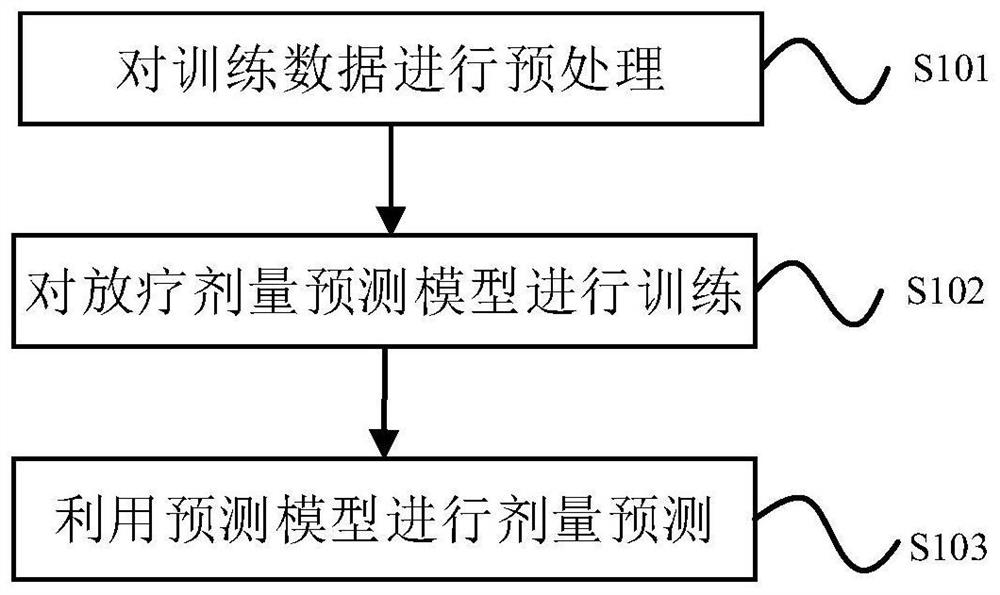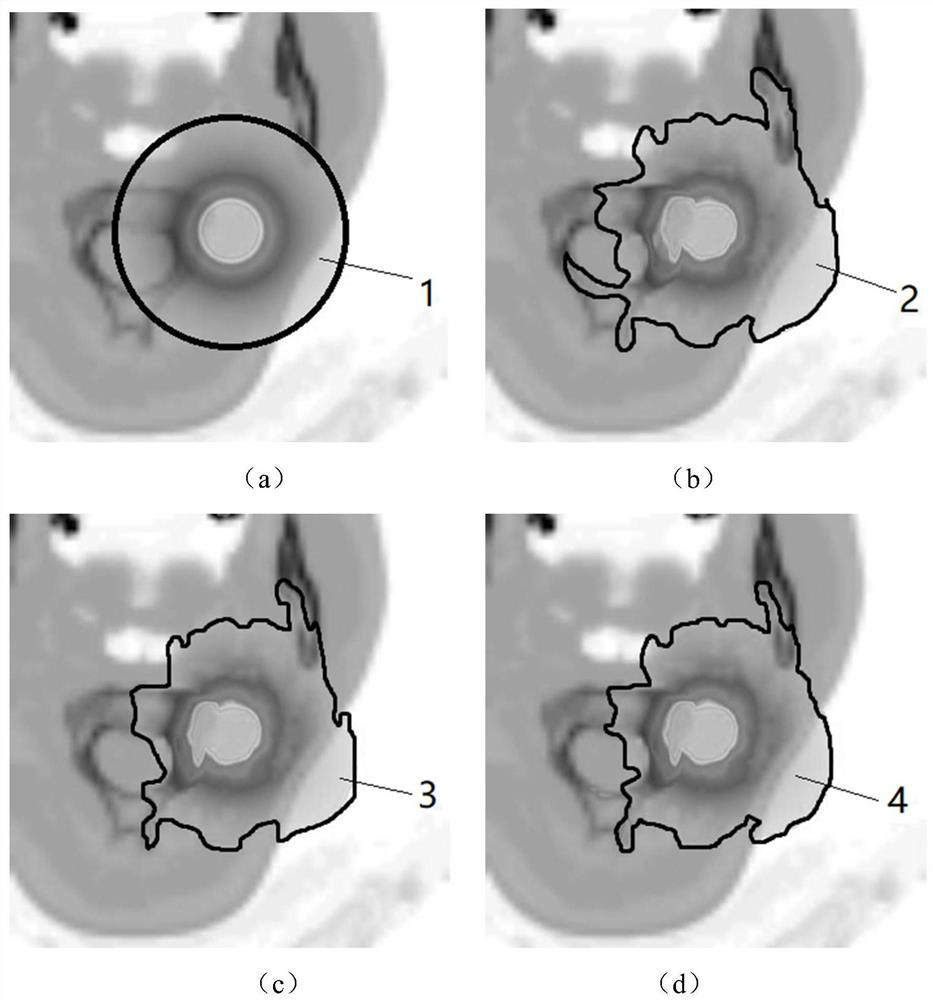# Radiotherapy dose prediction method and device

## A dose prediction and dose technology, applied in radiation therapy, X-ray/γ-ray/particle irradiation therapy, treatment, etc., can solve the problems of difficult dose calculation and optimization, long time, etc.

Active Publication Date: 2021-11-05
BEIHANG UNIV
7 Cites 0 Cited by

## AI-Extracted Technical Summary

### Problems solved by technology

However, Monte Carlo simulation takes a long time and is difficult to be directly applied in clinical practice for dose calculation and...
View more

### Method used

It should be explained that the position of the radioactive particles is modified several times, and when each position is modified, the dose distribution H and L are recalculated, which can expand the sample of training data, thereby improving the learning accuracy of the deep learning neural network.
 In an optional embodiment, the deep learning neural network is 3D U-Net. 3D U-Net has the characteristics of automatic feature extraction, extraction of three-dimensional space information, automatic optimization, etc., and is suitable for dose prediction. It includes an encoding stage that extr...
View more

## Abstract

The invention discloses a radiotherapy dose prediction method, which comprises the following steps of: preprocessing: establishing a patient model body according to the medical image of a patient; calculating the dose distribution H of radioactive particles in the patient model body by using a first calculation method of a radiotherapy dose; using a TG-43 method and a tissue heterogeneity correction method to obtain the dose distribution L of the radioactive particles in the patient model body; carrying out model training: taking multiple times of dose distribution L and the medical image of the patient as input, taking multiple times of dose distribution H as output, and sending into a deep learning neural network for training to obtain a radiotherapy dose prediction network; carrying out dose prediction: sending the medical image of any patient into the input end of the radiotherapy dose prediction network, and obtaining dose distribution L * by using the TG-43 method and the tissue heterogeneity correction method; and obtaining the dose distribution H *, which is predicted by the output end of the radiotherapy dose prediction network, of any one patient model body. According to the technical scheme disclosed by the invention, the technical effect of predicting the output result of a high-precision algorithm by using a low-precision algorithm is achieved.

Application Domain

Technology Topic

Dose predictionMedical physics +7

## Image

•••## Examples

• Experimental program(6)

### Example Embodiment

 Example 1:

### Example

 figure 1 It is a schematic diagram of a radiotherapy dose prediction method of the first embodiment of the present invention, such as figure 1 As shown, the method includes three steps as follows.
 Step S101: Preprocessing the training data. According to the patient's medical image, the patient's mold is established; the dose distribution h of the radiotherapy in the patient's mold is calculated using the radiotherapy dose first calculation method; the dose distribution L1 in a uniform water mold is calculated by the TG-43 method, and the tissue isoform The quality correction method correction is corrected to the dose distribution L1 to obtain the dose distribution L of the radioactive particles in the patient's mold; modify the position of the radioactive particles, and the dose distribution H and L are recalculated when modified each time.
 Illustratively, the patient's mold can be a three-dimensional data of a patient's medical image. In engineering practice, medical image data is two-dimensional data, and three-dimensional data is established by three-dimensional reconstruction methods.
 Exemplarily, the dose distribution obtained by the radioactive particles in the water mold is calculated using the TG-43 method:

 Where S k For the particle source air than the strength strength, λ is the dose rate constant, g L Particle source geometric function, g L The radial dose function, F is an anisotropic function, and these values ​​are given by the particle source manufacturer and TG-43. Then use the heterogeneous tissue correction factor (ICF) to correct:

 Where μ, μ ab The sum ρ is a linear attenuation coefficient, a linear absorption coefficient, and a density, which are calculated as follows: According to the medical imaging pixel value, the corresponding density and elements are converted to the corresponding density, such as the corresponding density and element, and then The corresponding linear attenuation coefficient and linear absorption coefficient are calculated according to NIST official website data. The subscript Het and W represent the media as heterogeneous tissues and water, respectively. k a K b For the built coefficient, it can be obtained from Monte Carlo. The particle dose distribution of the heterogeneous tissue correction coefficients by multiplying the uniform water mold calculated by the TG-43 method obtains the initial correction dose distribution:


### Example

 figure 2 A dose distribution of a radiotherapy dose prediction method for the first embodiment of the present invention. like figure 2 Distance figure 2 (a) is a schematic diagram of the dose distribution of the radioactive particles in the water mold; figure 2 (b) is figure 2 (a) Schematic diagram of dose distribution after dose distribution correction. Since the TG-43 method is directly obtained by radioactive particles in a uniform water mold, figure 2 The dose distribution 1 in (a) is a uniform circular shape, and the dose distribution after heterogeneous tissue correction is figure 2 The dose distribution 2 in (b) is shown.
 In an alternative embodiment, the radiotherapy dose first calculation method is Monte Carlo simulation method. Monte Carlo simulates the calculation dose distribution based on the physical process of particles in computer simulated substances, and has a high precision in the field of radiation therapy, but its calculation is less efficient, and the time required for single radioactive particle dose distribution is about 20. minute. figure 2 (c) is a schematic diagram of the dose distribution calculated by the Monte Carlo algorithm.
 It is to be described that the location of the radioactive particles is modified, and when the location is modified, the dose distribution H and L are recalculated, and the samples of the training data can be extended, thereby increasing the learning accuracy of the depth learning neural network.
 It is to be described that the radiotherapy dose first calculation method and TG-43 method are used to calculate the discharged dose distribution. The TG-43 method has the characteristics of high computational efficiency but low calculation accuracy. In contrast, the first calculation method has the characteristics of low calculation but high calculation accuracy. The deep learning neural network obtained by training is to quickly predict the H. Optionally, the patient's medical image uses a CT image.
Step S102: Training the radiotherapy dose prediction model. Specifically, the medical image of several dosage distributions L and the patient is input, with a number of dose distribution h, which is output, and the depth learning neural network training is delivered to the radiotherapy dose prediction network.
 In an alternative embodiment, the depth learning neural network is 3D U-NET. 3D U-NET has automatic extraction characteristics, extracting three-dimensional spatial information, automatic optimization, etc., suitable for dose prediction. It includes a coding phase extracted by the data layering of the input channel, and the decoding phase of the decoding phase required to reconstruct the output channel, the sampling phase and the sampling phase of the sampling phase and the anti-roll on the sampling stage are used. Skip connections Connect the output of the early convolutional block to the input of the backbone of the network. This reduces the size of the volume block, but reduces the redundancy inside the network, which helps to reduce the loss of information caused by the multi-scale structure of the network architecture.
 Step S103: Dose prediction using the prediction model. Specifically, according to the medical image of any patient, any patient body is established; the input end of the radiotherapy dose prediction network is sent to the medical image of any patient, and the TG-43 method and the tissue heterogeneous correction method are obtained. Dose distribution L *; obtains the dose distribution H * of any patient mold that is predicted by the radiotherapy dose predicting the network output.
 figure 2 (d) is a predicted dose distribution diagram. Exemplarily, such as figure 2 (d), the dose distribution 4 obtained by radiotherapy dose prediction network figure 2 (c) The dose distribution of the dose distribution obtained by the Monte Carlo Algorithm is better, thereby realizing the technical effect of using the low precision algorithm to predict the calculation results of high precision algorithm.

## PUM## Description & Claims & Application Information

We can also present the details of the Description, Claims and Application information to help users get a comprehensive understanding of the technical details of the patent, such as background art, summary of invention, brief description of drawings, description of embodiments, and other original content. On the other hand, users can also determine the specific scope of protection of the technology through the list of claims; as well as understand the changes in the life cycle of the technology with the presentation of the patent timeline. Login to view more.
Who we serve
• R&D Engineer
• R&D Manager
• IP Professional
Why Eureka